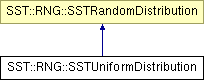# SST::RNG::SSTUniformDistribution Class Reference

Creates a Uniform distribution for use within SST. More...

`#include "sst/core/rng/uniform.h"`

Inheritance diagram for SST::RNG::SSTUniformDistribution:## Public Member Functions

SSTUniformDistribution (const uint32_t probsCount)
Creates an uniform distribution with a specific number of bins.
SSTUniformDistribution (const uint32_t probsCount, SSTRandom *baseDist)
Creates a Uniform distribution with a specific number of bins and user supplied random number generaotr.
~SSTUniformDistribution ()
Destroys the distribution and will delete locally allocated RNGs.
double getNextDouble ()
Gets the next (random) double value in the distribution.

## Protected Attributes

SSTRandombaseDistrib
Sets the base random number generator for the distribution.
bool deleteDistrib
Controls whether the base distribution should be deleted when this class is destructed.
uint32_t probCount
Count of discrete probabilities.

## Detailed Description

Creates a Uniform distribution for use within SST.

This distribution is the same across platforms and compilers.

## Constructor & Destructor Documentation

 SST::RNG::SSTUniformDistribution::SSTUniformDistribution ( const uint32_t probsCount ) ` [inline]`

Creates an uniform distribution with a specific number of bins.

Parameters:
 probsCount Number of probability bins in this distribution

References baseDistrib, and deleteDistrib.

 SST::RNG::SSTUniformDistribution::SSTUniformDistribution ( const uint32_t probsCount, SSTRandom * baseDist ) ` [inline]`

Creates a Uniform distribution with a specific number of bins and user supplied random number generaotr.

Parameters:
 probsCount Number of probability bins in the distribution baseDist The base random number generator to take the distribution from.

References baseDistrib, and deleteDistrib.

## Member Function Documentation

 double SST::RNG::SSTUniformDistribution::getNextDouble ( ) ` [inline, virtual]`

Gets the next (random) double value in the distribution.

Returns:
The next random double from the distribution, this is the double converted of the index where the probability is located

Implements SST::RNG::SSTRandomDistribution.

References baseDistrib, SST::RNG::SSTRandom::nextUniform(), and probCount.

The documentation for this class was generated from the following file:

Generated on 14 Sep 2015 for SST by1.6.1